# Tutorials

Learning molecular dynamics simulations can appear daunting at first. “What is a cutoff?” “How do I add water to my protein?” Questions can be so numerous that the beginner has no idea where to start! That’s why a large number of tutorials have been written for helping novice (and experienced!) users alike. The largest collection of VMD and NAMD tutorials can be found at the TCBG website, but a few are also highlighted here.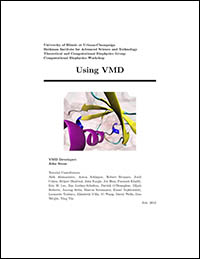VMD Tutorial
This tutorial addresses how to use the molecular visualization program VMD to view protein structures, build systems for simulation, and analyze results.NAMD Tutorial
This tutorial introduces the MD-simulation package NAMD as well as details much of the setup and input.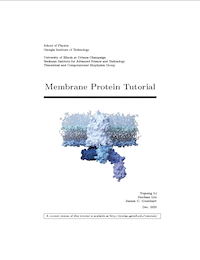Membrane Protein Tutorial
This tutorial goes through the steps of building a membrane-protein system using CHARMM-GUI and simulating it using NAMD. Associated files can be found here.

# Free Energy Tutorials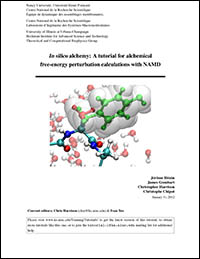FEP Tutorial
Alchemical free-energy perturbation allows one to change one species to another through non-physical intermediates, calculating the free energy of the process along the way.

• Instructions: pdf
• Required tutorial files: .tar.gz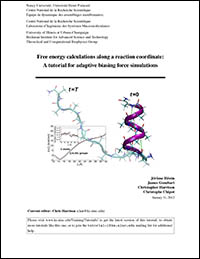ABF Tutorial
Adaptive-biasing forces (ABF) is a quasi-equilibrium method for determining the potential-of-mean force (PMF) along a chosen reaction coordinate.

• Instructions: pdf
• Required tutorial files: .tar.gz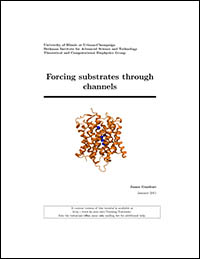Methods for calculating Potentials of Mean Force
Steered MD and free-energy techniques are applied to substrate translocation through the AmtB channel in this tutorial.

• Instructions: pdf
• Required tutorial files: .tar.gz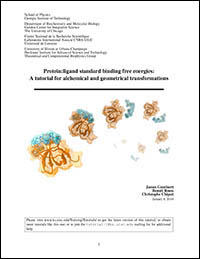Ligand Binding Tutorial
How to calculate accurate binding constants using a staged free-energy procedure is explained in detail.

• Instructions: pdf
• Required tutorial files: .tar.gz